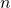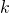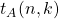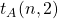# Partitions with k sizes from a set

A. David Christopher
Notes on Number Theory and Discrete Mathematics
Print ISSN 1310–5132, Online ISSN 2367–8275
Volume 28, 2022, Number 1, Pages 100—108
DOI: 10.7546/nntdm.2022.28.1.100-108

## Details

### Authors and affiliations

A. David ChristopherDepartment of Mathematics, The American College

### Abstract

Letandbe two positive integers and letbe a set of positive integers. We defineto be the number of partitions ofwith exactlysizes and parts in. As an implication of a variant of Newton’s product-sum identities we present a generating function for. Subsequently, we obtain a recurrence relation forand a divisor-sum expression for. Also, we present a bijective proof for the latter expression.

### Keywords

• Newton’s product-sum identities
• Size of a partition
• Recurrence relation

### 2020 Mathematics Subject Classification

• Primary 05A17
• Secondary 11P99

### References

1. Andrews, G. E. (1999). Stacked lattice boxes. Annals of Combinatorics, 3(2), 115–130.
2. Benyahia Tani, N., & Bouroubi, S. (2011). Enumeration of the partitions of an
integer into parts of a specified number of different sizes and especially two sizes.
Journal of Integer Sequences, 14, Article 11.3.6.
3. David Christopher, A. (2015). Partitions with fixed number of sizes. Journal of Integer Sequences, 18, Article 15.11.5.
4. Keith, W. J. (2017). Partitions into a small number of part sizes. International Journal of Number Theory, 13(1), 229–241.
5. Riordan, J. (1958). Introduction to Combinatorial Analysis, John Wiley & Sons, Inc., New York (1958).
6. Zeilberger, D. (1984). A combinatorial proof of Newton’s identities. Discrete Mathematics, 49(3), 319